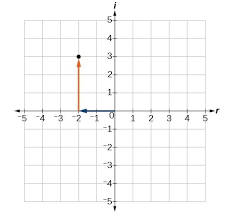# Which quadrant should the graph of -4 - 9i be in?

## Question:

Which quadrant should the graph of -4 - 9i be in?

## Complex Numbers:

Imaginary numbers were first thought to be a ridiculous notion. Descartes coined the term "imaginary numbers" as a derogatory name for this branch of mathematics. However, in time, imaginary numbers began to prove their worth.

Imaginary numbers are defined as the set of multiples of {eq}x {/eq} and {eq}i = \sqrt{-1} {/eq}, where {eq}x {/eq} is any element of the set of real numbers.

Complex numbers are sums of a real number and an imaginary number, of the form {eq}a + bi {/eq}, where {eq}i {/eq} is the imaginary unit. Just as real numbers can be plotted on a number line, imaginary numbers can be plotted too, but they require a 2-dimensional space, with one real axis and one imaginary axis (the real axis has units of 1, and the imaginary axis has units of {eq}i {/eq}).With this style of plot in mind, we can easily determine the quadrant of the given complex number. The real part and imaginary part are both negative, so -4 - 9i must be in the 3rd quadrant (bottom left).